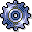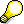Costing DatesUse

The following dates are relevant for a material cost estimate and a costing run:

• Quantity structure date
• Valuation date
• Costing date from/to

You can define a date control ID in Customizing for Product Cost Planning that determines the following:

• Which dates are proposed
• Whether the user can change the proposed dates

The date control ID is assigned to a costing variant.

Features

The quantity structure date determines how the system selects a valid quantity structure for the cost estimate. Based on this date, a BOM and a routing are selected, exploded and costed. The quantity structure date also determines which additive cost estimate is selected.

The valuation date determines how the system searches for valid data to calculate the following prices:

• Prices for stock materials from the material master record
• Activity prices for activity types from cost center planning
• Prices for externally-procured materials from purchasing
• Prices for externally-processed operations from purchasing

You set the validity period of the cost estimate with the Costing date from and Costing date to indicators.

You use the costing type in Customizing for Product Cost Planning to specify whether cost estimates are updated in the database with a date. You have the following options:

• Without date
• With date
• With start of period

For costing types which have the With date or With start of period indicator set, the system uses the date or period start that is entered in the Costing date from field.

• For the standard cost estimate, the With start of period indicator must be set. This means that the period and the fiscal year of costing are parts of the costing key in the database. This ensures that
• Only one standard cost estimate can be stored within a period
• Only this standard cost estimate can be transferred into the material master record as the standard price
• For the modified standard cost estimate, the With start of period indicator is automatically set. If you want to save several modified standard cost estimates for the same material in one period, set the With date indicator. The date of the cost estimate is saved as part of the costing key in the database.
• For the current cost estimate, the Without date indicator is automatically set. The date is not included in the costing key.

Depending on whether you carry out costing manually or automatically, you must remember the following:

• If costing is carried out automatically, the Costing date from applies. The Costing date to is just for informational purposes.

You cannot create more than one cost estimate with the same validity period, because cost estimates with the same Costing date from would overwrite one another.

On the other hand, you can create automatic cost estimates whose validity periods overlap. In this case, the entries in the field Costing date from are different.

• If costing is carried out manually, then both the Costing date from and the Costing date to apply.

The validity periods of the additive cost estimates must not overlap.The validity period of the cost estimate is also relevant for Cost Object Controlling. The results of the standard cost estimate are used in the calculation of:

• Variance calculation
• Scrap calculation
• Work in process calculation

If the standard cost estimate is not valid on the date on which these functions are to be performed, the system issues an error message.